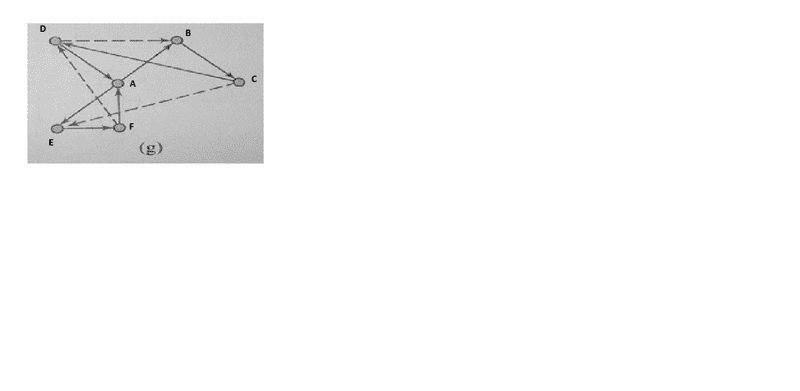# Graph: difference between cycle & circuit

• Engineering
• zak100

## Homework Statement

What is the difference between a cycle & a circuit? (Definition not reqd, guidance reqd with the figure attached)

No

## The Attempt at a Solution

See the attached figure. According to this figure the walk AB, BC, CE, EF, FD, DA represents a cycle or not because we have a repeated vertex B?? (in my view its not a cycle because we have a repeated vertex in the middle).However it represents a circuit because there is no repeated edge.
Some body please guide me whether i am correct or not & give an example of cycle from the figure. Sorry for the picture not fitting with size. I have created it on paint & then saved it but i don't know how to get rid of extra white space.

Zulfi.Both cycles and circuits are closed paths, meaning they end at the same vertex as where they started.
For a circuit, vertices may be repeat but not edges.
For a cycle, neither edges nor vertices may repeat (except that the start vertex is the same as the finish vertex).
Hence all cycles are circuits, but not vice versa.

The path listed above is a cycle (and hence also a circuit).
Why do you think it has a repeated vertex? As I read your post it goes ABCEFDA, so there are no repeated vertices (there are six vertices and six edges).

An example of a circuit that is not a cycle would be a figure eight, such as DBCDAFD

Hi,